# Chapter 2c: Neutralization and titration

Neutralization

A neutralization reaction is the reaction occurring between an acid and a base forming a salt and water.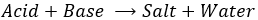Technically, the neutralisation is not a one step reaction in the sense that all the actions are not done simultaneously but step by step. The fist step is the dissociation of the acid and base from their conjugate species. The second step is the formation of the salt from the conjugate species and of water from H3O+ and OH. For example, NaOH is neutralised by HCl to form NaCl (cooking salt) and water.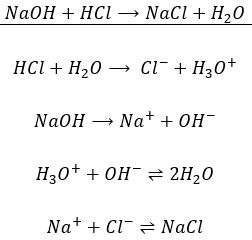The first equation is indeed the addition of the 4 equations underneath. If a compound is found at both sides of the equation, as the ionic species, we don’t write them in the mean equation. Note that all the molecules of  Na+ and Cl–

are not reacting to give NaCl. There is here an equilibrium between the species in solution and the salt precipitating. This equilibrium is to be seen in the section of Dissolution.

The neutralization point is reached when the quantity of acid and base put in solution are equal. All the reactants are then consumed and for this reaction the pH is neutral, i.e. pH=7.

Titration of strong acids/bases

Titration is a method used to determine the concentration of a compound through its neutralisation. For example, the concentration of a solution of HCl can be determined by the addition of a solution of NaOH. The concentration of the titrating solution is known. As we are in presence of a strong acid (HCl) and a strong base (NaOH), both of them completely dissociate in solution. The concentration of proton is initially equivalent to the concentration of Cl. In the other solution, the concentration of OH and of Na+ is equivalent of the known concentration of NaOH.

To obtain a neutral pH, the number of protons na (in moles) has to be equal to the number of OH, nb (in moles). In other words, neutralisation, or equivalence, is reached when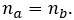The number of moles of a compound in a solution is simply the concentration of this species multiplied by the volume of the solution: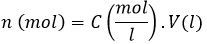From the two previous relations, we can find the initial concentration of acid that we wanted to determine: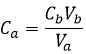As an example, if 20ml of NaOH 0.01M was required to neutralise a volume of 10ml of the HCl solution, [HCl]=0.02M.

In laboratory, titrations are performed as follow:

A flask containing a given volume of the unknown solution is placed on a magnetic mixer. The magnetic chip is placed in the solution to mix it continuously during the experiment. To be able to observe the neutralisation process, two droplets of pH indicator are added to the solution. Several indicators can be used to observe the passage through pH=7. Here, we will use bromothymol blue the color of which changes from yellow (pH<6.0) to blue (pH>7.6). At pH=7, the color is green. Considering the previous example, our solution is thus yellow. We can already say that the solution is acidic and that its pH is smaller than 6.

The neutralising solution, NaOH 0.01M in our case, fills a burette placed a few centimetres above the other solution. There is no need to add color indicator in this solution.  Manipulating carefully the burette, NaOH is slowly added to the acid solution. One can read the consumed volume of base on graduations of the burette. When pH approaches 6, one can see the base droplets turning blue while mixing in the solution. Not knowing at all the concentration of the acid, it is convenient to perform a fast experiment to determine an approximate volume for the neutralisation and to perform a second experiment, only going slowly at the approach of this volume. Typically, the color will change from yellow to green or directly to blue at the fall of one droplet. The precision of this experiment is thus limited to the precision of the burette. Generally, the volume of a droplet is the half of a graduation.

The titration curve of this example is shown next.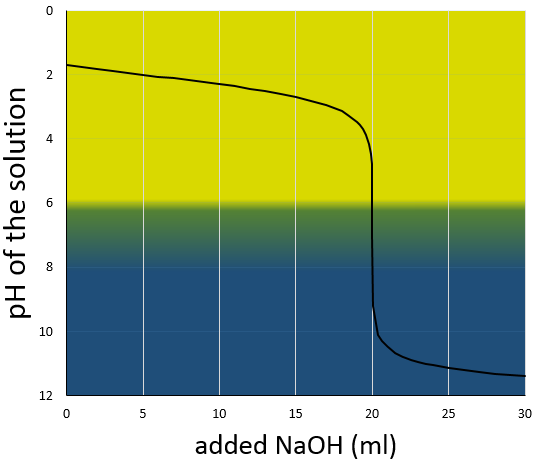Remember that the pH is the logarithm of the concentration of protons while the addition of the base is linear, and that the volume of solution increases with the addition of the base (don’t forget that point when choosing the volume of the flask). As we can see, the pH variation is mainly focused at the vicinity of the neutralisation point. At 20ml, pH=7 but at 19.95ml (one less droplet approximately, for a 50ml burette), pH=4.78 and at 20.05ml, pH=9.22.

We can also find the equivalence point by tracing the tangents of the curve in the acid region and in the base region. Those two tangents are parallel. When we trace the line equidistant to those two line, it meets the equivalence point.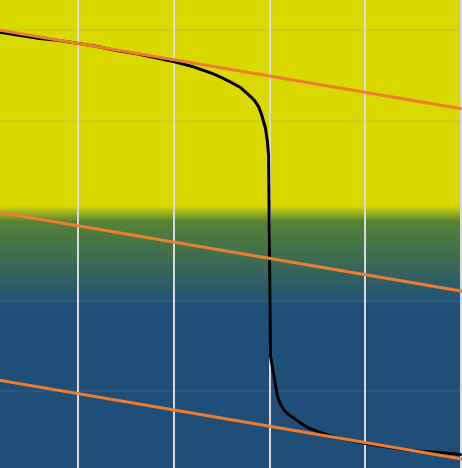Titration of weak acids/bases

The titration of a weak acid is done using a strong base and follows the same principle. Lets perform the titration of the acetic acid. The reaction is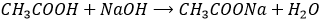This reaction is complete. Before the titration, the pH of the acetic acid solution is simply given by the relation for a weak acid saw in the previous section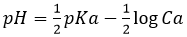As for the strong acids, the equivalence is reached whenHowever, the pH at the equivalence is not neutral but basic. Indeed, the conjugate species of a strong base/acid is inert but the conjugate species of a weak acid/base is itself a weak base/acid: pKa + pKb = 14. All the acetic acid and NaOH are consumed but acetate has been produced and it is a weak base.

Before the equivalence, the pH depends on the quantity of weak acid and of its conjugate base: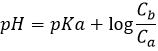This melange between a weak acid and its conjugate base is called a buffer solution because the addition of a strong base or acid does not modify sensibly the pH of the solution. Buffer solutions are very important for living species to resist to sudden variations of the environment. An example of buffer solution is our stomach. No matter what we eat or drink, its pH is unaffected (approximatively) so that the job can be done. In our whole body, enzymes are effective in a given area of pH and to keep them working, the pH has to be regulated through buffer solutions.

The semi-equivalence is the point when there is as much CH3COOH that CH3COO(Ca=Cb). At this point, pH=pKa. To reach the semi-equivalence point, the added volume of base is the half of the volume to obtain the equivalence.

At the equivalence, pH is given by the amount of acetate in the solution (formula for a weak base). This amount is equal to the one of NaOH added to the solution.

After the equivalence point, pH is given by the quantity of NaOH in the solution.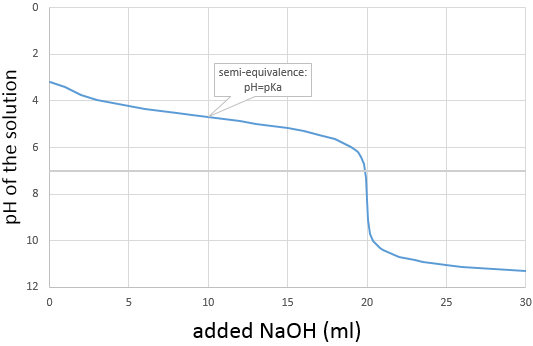Titration of a polyacid

Considering a polyacid HnA (a concrete example will be given later) with different enough pKa’s, the neutralizations of the different forms of the acid are successive: The OH will first neutralize the protons released by HnA and then the protons released by Hn-1A, etc.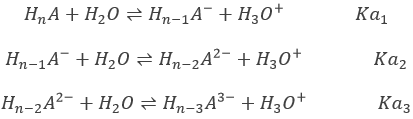The initial pH of the solution is the pH of HnA. The concentrations of the subsequent acids are negligible. HnA may be a strong acid or a weak acid.

At the first equivalence point, Hn-1A is the main species in solution. It is an amphoteric species, i.e. it can accept or donate protons. The pH is thus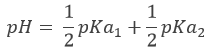At the next semi-equivalence point, [Hn-1A]=[ Hn-2A2-] and we are in a buffer solution. Remember that the pH in buffer solutions is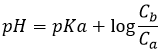The pH is thus pH=pKa2. Note that if the initial acid is a weak acid, the same is true for the first semi-equivalence point, i.e. pH=pKa1.

It is interesting to note that for those specific values, pH does not depend on concentrations.

Let’s take the case of H3PO4 as an example. Its pKa’s are very different from each other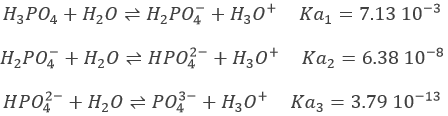The neutralisations are successive and we can thus find the specific points (semi-equivalences and equivalences) determined above.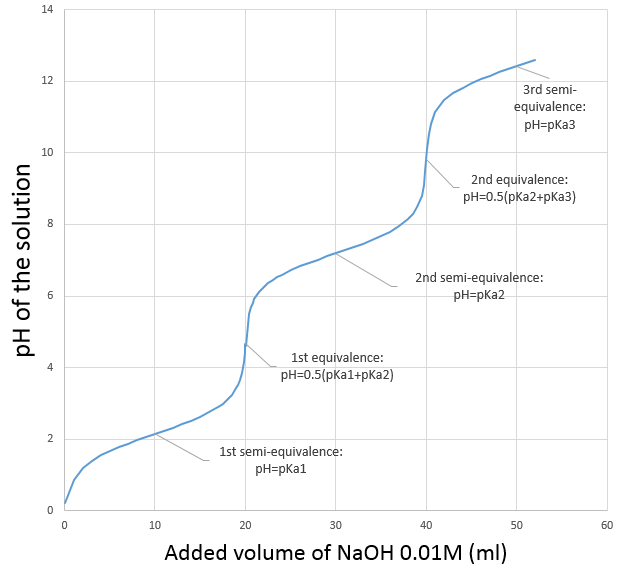H3PO4 is a weak acid. The initial pH of the solution is thus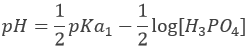Before the equivalence, H3PO4 and H2PO4 are in solution. This buffer solution has a pH of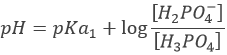With pH=pKa1 when [H3PO4]=[H2PO4], at the semi-equivalence.

At the first equivalence, H2PO4 is the main species in solution. It is an amphoteric species and the pH is thusAfter the first equivalence, H2PO4 and HPO42- are in solution. This is again a buffer solution.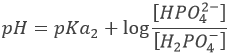With pH=pKa2 when [H2PO4]=[HPO42-], i.e. at the second semi-equivalence. At the second equivalence point, HPO42- is the main species in solution and is amphoteric.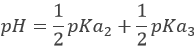After this equivalence, the solution is again a buffer solution.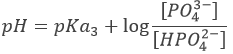With pH=pKa3 when [HPO42-]=[PO43-], i.e. at the third semi-equivalence.

At the third equivalence, PO43- is the main species in solution. This is not an amphoteric species but a weak base. The pH should be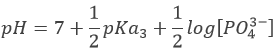However, Ka3 is very close to Kw. Protons released by water are in competition with protons from HPO42- and the prediction does not stand anymore. To calculate the pH, we need to go back to the full composition of the solution and then solve the equation. This will not be done here.

Exercices

1. What is the color of bromothymol blue in a 20ml solution of NaOH 0.005M? If we add 10ml, 20ml or 30ml of HCl 0.005M?
2. What is the color of bromothymol blue in a 20ml solution of H3PO4 0.1M? If we add 10ml, 20ml or 30ml of NaOH 0.1M?